# Why Multiple Representations are Essential

Our calculus class on Tuesday was investigating derivatives of functions involving logarithms, particularly functions including natural logs. To begin our notes set, I worked a problem from algebra to help students remember how we use inverse operations to solve equations. I took direction from the students and solved the problem below in the following way.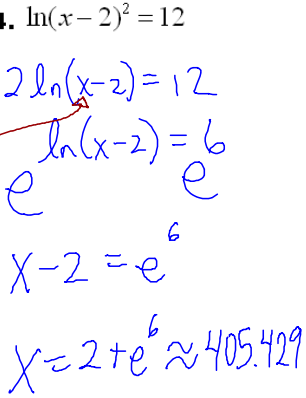I knew what would happen in advance - that students would simply go through the motions and apply algebraic properties to isolate x and move on. On a yearly basis, this is one of a set of problems I like to use to demonstrate to students the value of multiple representations. I then showed them the graph of the equation and how we could solve for the intersection of the logarithmic function and the constant function 12.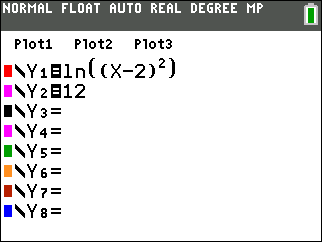We had an interesting discussion about the viewing window. Most students jumped all over "ZOOM-6", or Zoom --> Standard, the viewing window with x-axis and y-axis spanning the values -10 to 10 with units (tick marks) of 1.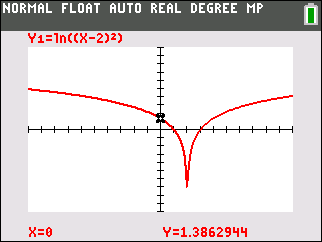Hmmmmm.... where's the 12? It's off the screen, of course!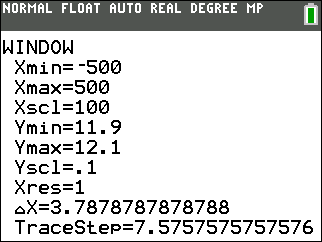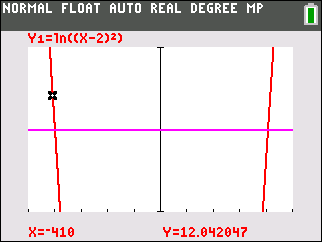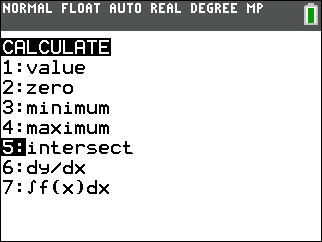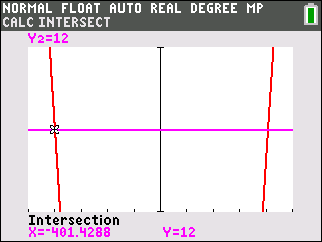After challenging their solution with the graphical argument, we decided to revisit our algebraic approach.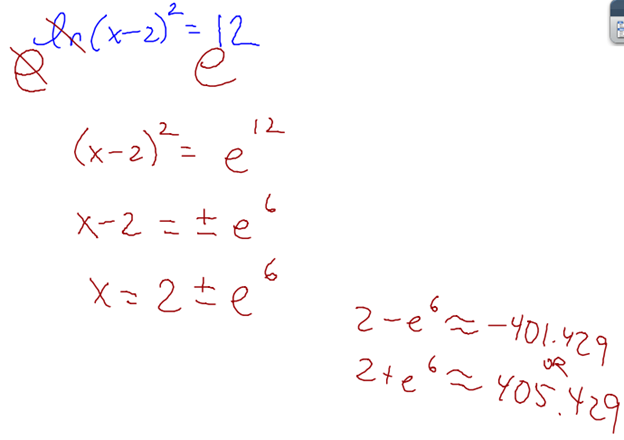This was a powerful way to convince the students that the exponent 2 acting on the quantity x - 2 has the effect of making the result positive, and it drove home the idea that we must consider the definition of absolute value when dealing with squares and square roots.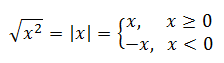We also had a good discussion about the original expression and whether the second power was acting on the quantity (x - 2), such as ln[ (x-2)*(x-2) ], or if it mattered if we consider ln[x-2]*ln[x-2].

Students often believe the plastic genie - the graphing calculator - knows all. I really enjoy the days in math class where I can show examples that disconfirm this student perception.

# Implicit Differentiation FRQs • 4 Comments

Implicit differentiation really helps my students understand why we can't just arbitrarily slap a prime on a function when we differentiate, that the variable we differentiate with respect to matters. Discussing the differences between y' and dy/dx, or P' and dP/dt helps facilitate later topics too, like related rates and optimization.

We had our second calculus class of the semester today. We spend part of the time going over results from our fall semester final exam. We also worked on a free response question from an old AP exam, 2000 AB #5 that mirrored another old AP question I have on our fall final. The question we worked today is below.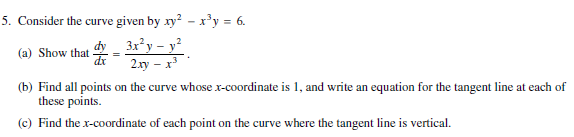We had some good conversation about what it means to show the expression given in part a for dy/dx corresponds to the given implicit curve. After doing the implicit differentiation, we ended up with this: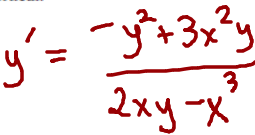I argued with the students our job wasn't quite done because the expression to the right of the equals sign does not match exactly with the original expression given in part a. I then wrote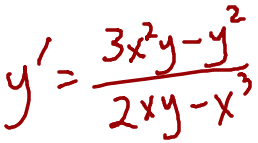And declared part a "done." A student challenged me, saying the y' did not match the left hand side of the equals sign of the original in part a (dy/dx). Great observation. I told the students if we are being precise, we should also go a step further and demonstrate we know y' and dy/dx are equivalent by writing dy/dx on the left. While this might seem like splitting hairs, I want my students to know attention to detail matters.

Part b came and went without much trouble. We found the two points on the curve whose x-coordinate is 1 and constructed the tangent line equations.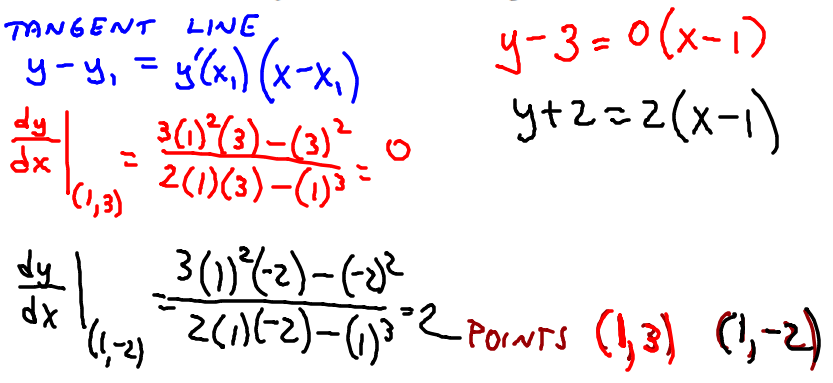Then came part c. As the students were working part c, I went to Wolfram Alpha and created a graph of the implicit curve.I wasn't too concerned with the restrictions on the scale or viewing window. As we worked through part c together, the students understood why we needed to set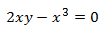because this would retrieve an undefined result for dy/dx.

The algebra we did looked like this: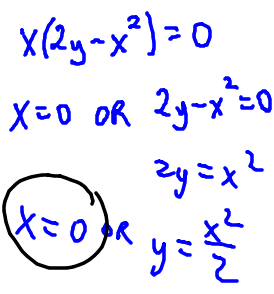The reason x = 0 is circled is because I forgot to verify at the end of the problem that x=0 is not a valid answer. If we try to directly substitute x = 0 into xy2 – x3y = 6, we get 0 = 6 which is nonsense. The substitution confirms x cannot be zero on the graph despite the fact we must infer the asymptote on the graph.

Here's another screenshot of the last slide I wrote during class: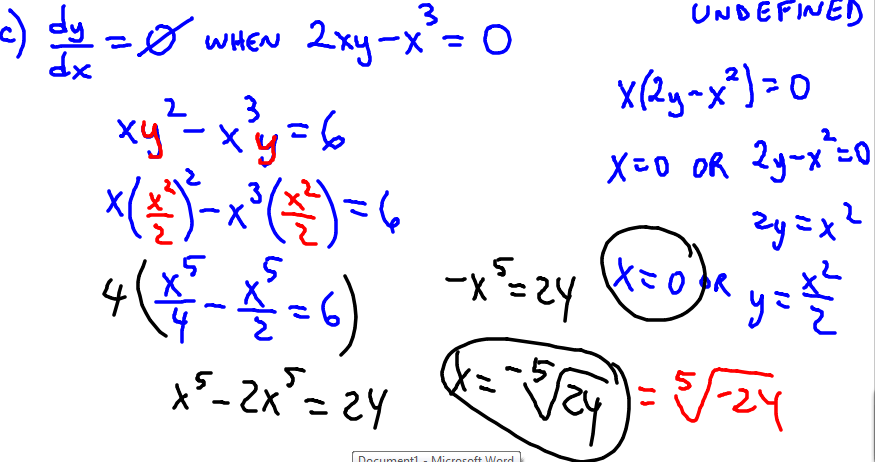I wrote -x5 = 24 and then asked how to isolate the x. I then wrote the solution (in black) above. The students were having trouble reconciling that the black value is equivalent to the red value. I referenced even and odd roots as the reason why I could pull the negative out of the radical (going from red to black).

HERE'S WHAT I WISH I HAD WRITTEN ON THE BOARD DURING CLASS...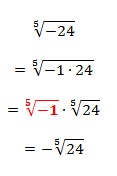I could sense the unease in the room around the solution, but I couldn't quite put my finger on it. After reflecting on this, I think I know why the kids seemed to disengage a bit. I have a lot of experience with negatives and roots and so on, so the simplification was not a stretch for me based on my experience. But the kids needed to see the work above, to reference the algebraic rules they know, to understand why it is permissible to move the negative out in front. I struggled to diagnose the students' need for this explanation during class. In hindsight, it explains why the students seemed to disengage and why my spider sense started tingling.

This post is really a reference for myself later, to remind me to think carefully on anticipated errors and what I can do to help students reconcile quantities that are numerically equivalent but not obviously numerically equivalent.

# Combining Like Terms and Teaching Factoring

While lesson planning for calculus class, I was thinking this morning about common mistakes students make during simplification. Teaching students how to combine like terms can be a challenge. I'd like to share how I approach teaching combine like terms and factoring. Consider the Algebra 1 exercise below.

Textbook instructions:
"Simplify the following expressions. Use the Distributive Property if needed."

Problem: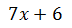How often do we see struggling students claim the above binomial is equivalent to 13x? We can quickly diagnose the misconception the student has... the student saw the addition symbol and combined the integers 7 and 6 as they did in their youth. The not-so-helpful hint "use the Distributive Property if needed" may distract the student from what they are being asked to do. I would pose the exercise in a different way.

Modified instructions:
"Simplify."

Problem:Note: Some may argue writing "simplify if possible" would be better since we may encounter some problems like this one where there really isn't any 'work' to be done. Instead of writing "not possible," I would rather see my students recognize the quantities 7x and 6 are relatively prime (with GCF of 1) because the value of x is unknown. To facilitate the teaching of factoring later in algebra, the student must also recognize when to "stop." Students will often ask, "How do I know when I can't factor it any further?" I like to revisit the definitions of prime and composite to address this notion of knowing when to stop.

I have my students write the following: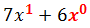Physical models like algebra tiles are one way to approach modeling the reason why we cannot combine 7x and 6. Let's look at another approach as to why we should not combine 7x and 6. Here's a contrived exchange between a student and a teacher that looks like how I approach this in my classroom verbally.

Teacher: What is the exponent on the x? <points to the x in 7x>

Student: Zero!

Teacher: If the exponent were a zero, we know any nonzero number to the zero power is 1. Then we would be multiplying the 7 by a 1, and we know multiplying any number by 1 does not affect the number's value. Also, we use zero to represent 'none.' So, if the exponent were a zero, then there would be 'no' x.

Student: Then the power must be a 1.

Teacher: That makes sense. What is the power of x on the 6? <points to 6>

Student: There's no x there!

Teacher: Would it be legal to draw a  ghost x0 to mean there is no x there?

Student: Okay.

Teacher: On page 89 of our algebra text, the author states "3x and 5x are like terms because they contain the same form of the variable x." The author then says 3x + 5x = 8x. What do you think the author means by 'same form'?

Student: The powers of x match in both.

Teacher: If that's true, then is it possible to combine 7x and 6 using addition or subtraction?

Student: No.

Then the curtains fall. The teacher and students move on to another problem. But let's take a second look. We still need a convincing argument to demonstrate the expressions 7x and 6 cannot be combined. Let's look at some specific cases.Suppose x = 1. Then the student is correct, since 7(1) + 6 = 13. But this substitution does not allow x to vary freely. Another way we can convince the student we cannot combine 7x and 6 would be to use a graph.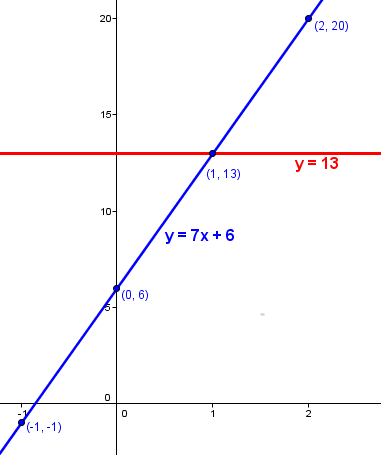The fact that the red line y = 13 and the blue line y = 7x + 6 is pretty convincing evidence the two quantities 13 and 7x + 6 are not the same for any x value other than 1, the intersection point on the graph. We can counsel the student that y = 13 is constant while y = 7x + 6, the line with slope 7 and y-intercept 6, depends on x. Let's consider another special case.

Suppose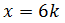for some integer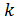. We can look at some specific cases to help our thinking about the consequences of this selection for x.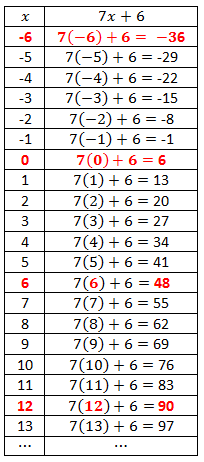We can now discuss the earlier statement, "Use the Distributive Property if needed." If we consider the case where x is an integer multiple of 6, then we have7k and 1 are not like terms, just as 7x and 6 are not like terms. 7k + 1 only equals 8 if k = 1. Why even think this way? Because we can tie the original problem back to factoring.I tell my students factoring is like playing tennis. Metaphorically speaking, we know a volley is over with in tennis based on what the ball does. We know we are 'done' factoring when we have an expression made of prime factors. I tell my students factoring is essentially a two step process:

Step 1. Greatest Common Factor (GCF)

Step 2. Depends on terms that remain. Applying a possible strategy may involve the whole collection of terms or a subset of the terms in the problem.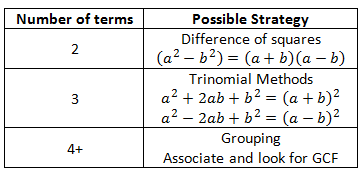We volley back and forth - do both steps - until we obtain expressions that are prime or constants that can be written as the product of primes. For instance, in the problem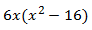, we could rewrite 6 as the product of 2 and 3... but the binomial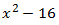can be rewritten as the product of prime linear factors x + 4 and x - 4.

Why do we consider x + 4 prime? Since the value of x varies, if we only consider integer values of x, then x + 4 may give a composite number or a prime number. Since we do not know the value of x, we think of x + 4 more conservatively as prime.

It does not take much to convince students that all numbers are divisible by 1. I insist my students look for a GCF every time, even in trivial problems, and identity the GCF is 1, or more specifically,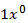. I try to emphasize that when we factor expressions with multiple terms, we look to factor out the "lowest" power the variable.

If we try and factor the original problem using this approach, we have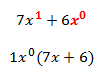since GCF(7, 6) = 1 and GCF(x, x^0) = x^0 = 1. This approach is particularly useful for later work in calculus, specifically when taking derivatives of expressions. My calculus students sometimes struggle with reconciling their solutions with the answer the text provides because they may not totally understand the factoring necessary for simplification. Students stumble when asked to find the GCF of the expression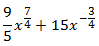. If students are accustomed to identifying the GCF, then problems of this type aren't as troublesome.